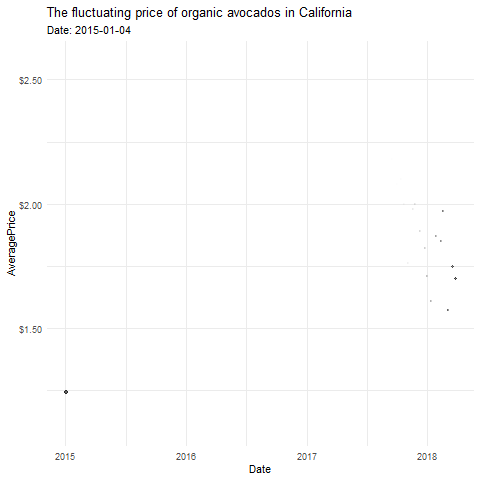# gganimate: A Lesson with AvocadosAnimating your ggplots may sound daunting. However, you have to add a line or two extra of code and you have an animation! `gganimate` makes animation quite accessible for users of ggplot.

A cheat sheet for what we’ll cover today:

• Types of Transitions
• transition_reveal() (good for mainly geom_line)
• transition_states() (good for geom_point, geom_bar, geom_col, etc)
• splits up plot data by a discrete variable and animates between the different states.
• transition_time() (geom_point)
• Instead of transitioning over a discrete variable, the transition occurs over a continuous variable (Time)
• Some extras
• This shadow lets you show the raw data behind the current frame. Both past and/or future raw data can be shown and styled as you want.
• instead of leaving a more permanent mark, wake leaves a tail to show direction, but only reveals the past few states
• view_follow(fixed_y = TRUE)
• controls how your viewport changes as the transition occurs
• `anim_save()`
• It works much like `ggsave()` from ggplot2 and automatically grabs the last rendered animation if you do not specify one directly.

Let’s load back up our data from the previous lessons on R by Adriana Picoral (picoral.github.io/resbaz_intro_to_r/parti.html) and from Kathryn Busby on ggplot2. I’ll name the dataframe `avocado` because I can’t remember what the other instructors named their data. We will also load our packages here.

``````library(tidyverse)
# install.packages("gganimate")
library(gganimate)
# install.packages("scales")
library(scales)

Let’s explore our data a little bit..

``````glimpse(avocado)
class(avocado\$Date) #make sure `Date` is actually a date type
unique(avocado\$region)# what type of regions are included here?``````

You’ll notice that our region variable is kind of all over the place. Because I’ve reviewed this before, I know we need to separate out the US level, states, regions, and cities so our graphs are on the same level.

``````avocado_us <- avocado %>% filter(region == "TotalUS")
states <- c("California")
regions <- c("West","Southeast","SouthCentral","Plains","Northeast","Midsouth","GreatLakes","WestTexNewMexico","NorthernNewEngland")

We’re finally ready to make some plots, and then build the animation into these plots.

## transition_reveal()

This type of transition is the simplest and acts like a piece of paper is being removed from left to right over the top of the graph to slowly reveal the result. That’s how I think about it, at least. This assume that your x axis is also what is included inside your statement transition_reveal().

For this, let’s first build a static line plot that has date on the x-axis. Looking through the data, we could use `AveragePrice` or `Total Volume` on the y axis, and we could disaggregate by region, size of avocado, or type (organic versus conventional).

Let’s stick to the totalUS aggregation dataset we made (avocado_us) and look at the average price of conventional and organic avocados over time.

``````ggplot(data = avocado_us,
mapping = aes(x = Date, y = AveragePrice, color = type)) +
geom_line()``````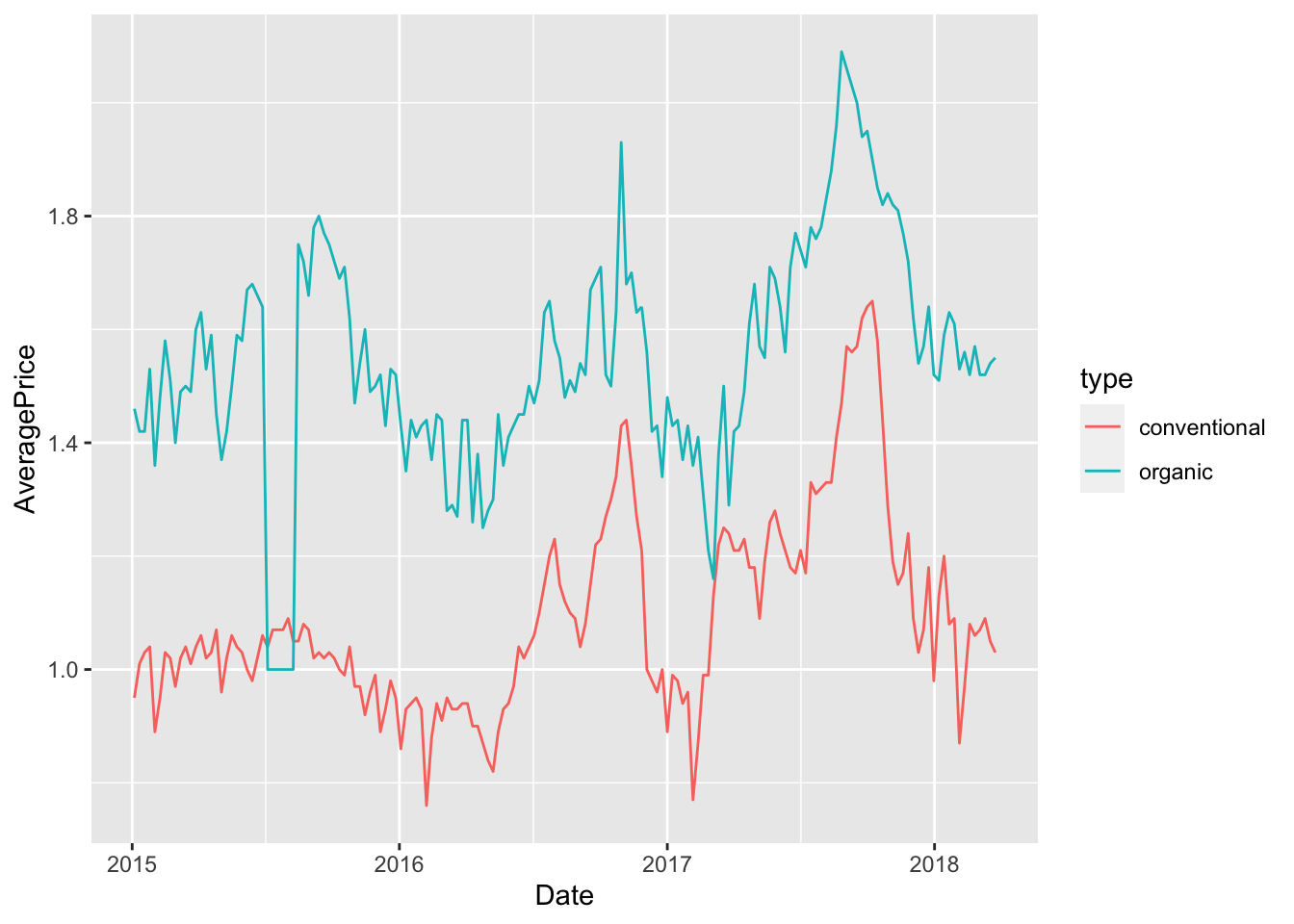If we feel good on time, we can make a few adjustments to the plot before animating it.

``````ggplot(data = avocado_us,
mapping = aes(x = Date, y = AveragePrice, color = type)) +
geom_line() +
scale_y_continuous(labels = scales::dollar_format()) +  # format that y axis!
scale_color_manual(values= c("darkgreen", "darkolivegreen3")) +
theme_minimal() +
labs(title = "Average Price of US Avocados",
caption = "Source: Kaggle")``````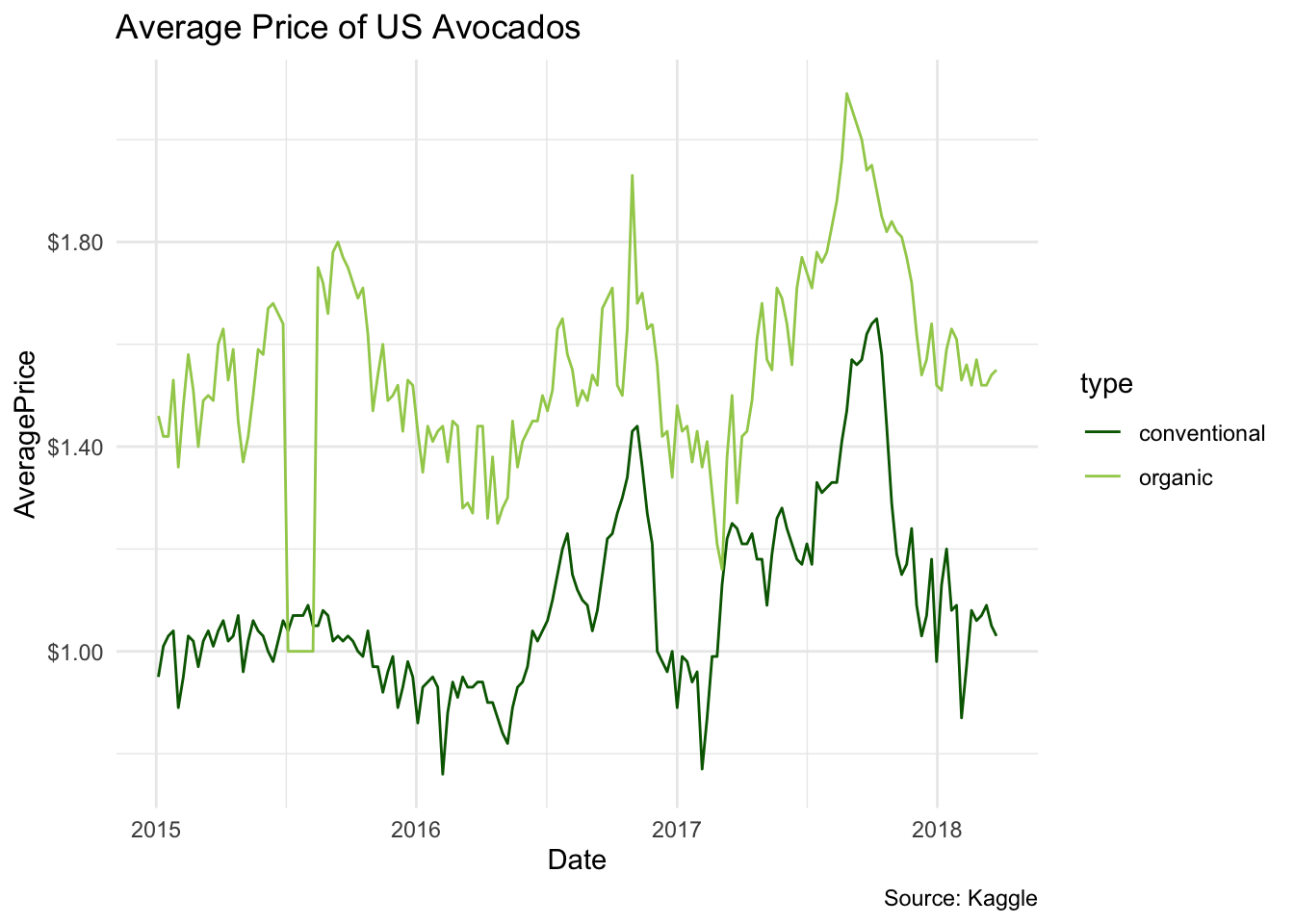This looks a lot better. I one what happened the summer of 2015! Now let’s animate this. The key to this animation is `transition_reveal()`. Inside of the function, we can write out x axis variable. While it will take a few moments to render, you should see an animated plot in your plots pane.

``````ggplot(data = avocado_us,
mapping = aes(x = Date, y = AveragePrice, color = type)) +
geom_line() +
scale_y_continuous(labels = scales::dollar_format()) +  # format that y axis!
scale_color_manual(values= c("darkgreen", "darkolivegreen3")) +
theme_minimal() +
labs(title = "Average Price of US Avocados",
caption = "Source: Kaggle") +
transition_reveal(Date)``````

Let’s also save this, since each time we run the code it takes some time.

``anim_save(filename = "type_reveal.gif")``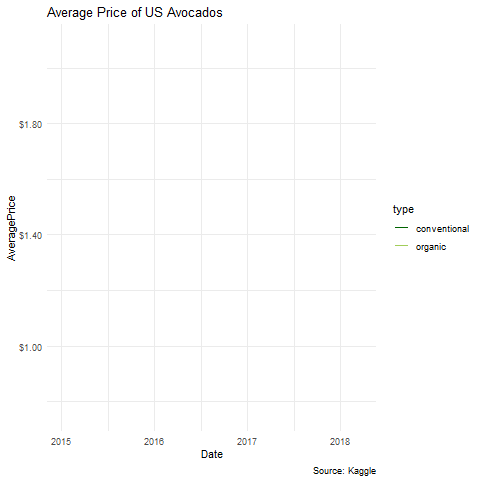### Challenge

Take a few minutes to try and plot the changes in total volume of organic avocados across time for the different regions of the USA.

## transition_time()

Transition time creates new “layers” of the animation over a continous variable, usually time (i’ve never seen an exception to that). While this works best with geom_point, there’s many other options you can play around with.

Let’s use two continous variables to plot this. Let’s see how well price explains the volume sold of avocados for non-organic avocados (though, it’s been awhile since I took Econ101). Let’s do this for the different cities in the US, omitting states and regions.

``````avocado_cities_filtered <- avocado_cities %>%
filter(type == "conventional",
Date > as.Date("2018-01-01"))
mapping = aes(x = AveragePrice, y = `Total Volume`, color = region)) +
geom_point()``````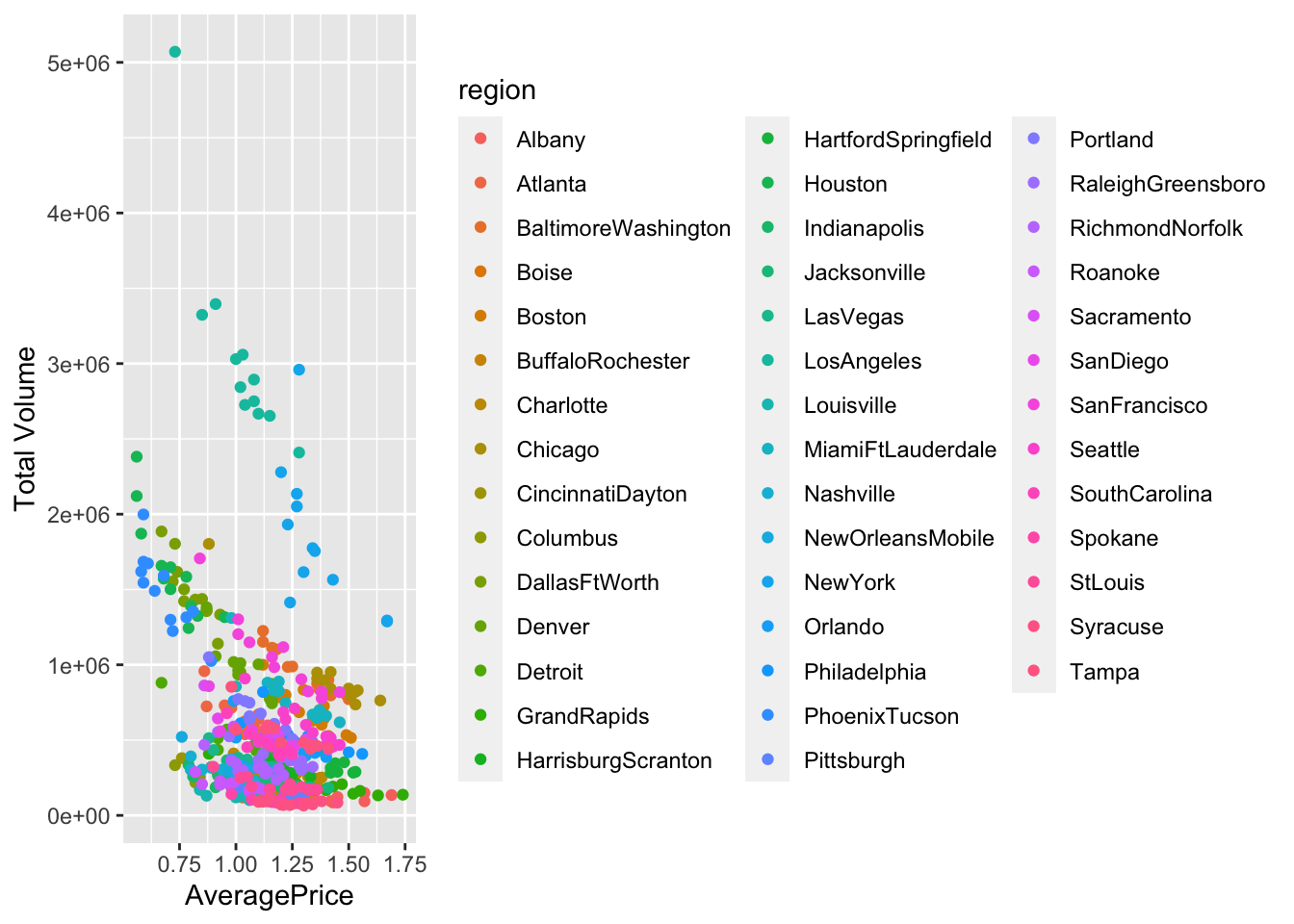That legend is really going to get in the way. Let’s remove it and customize the circles before animating.

``````ggplot(data = avocado_cities_filtered,
mapping = aes(x = AveragePrice, y = `Total Volume`, color = region)) +
scale_y_continuous(labels = scales::comma_format()) +
scale_x_continuous(labels = scales::dollar_format()) +
geom_point(aes(size = `Total Volume`), alpha = .6) +
theme_minimal() +
theme(legend.position = "none") +
labs(title = "Avocados sold by price and city") ``````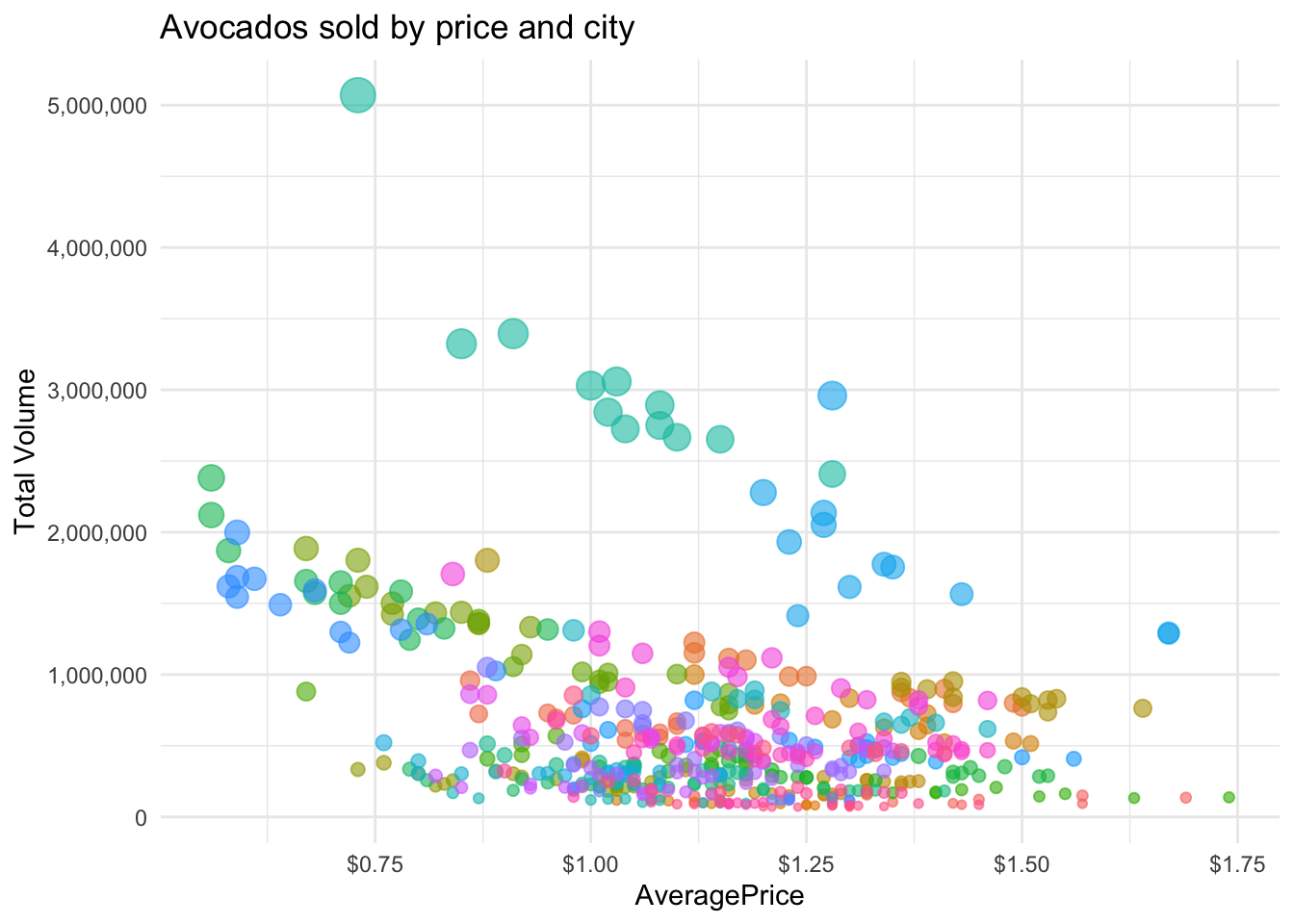In practice, the animation is basically layering a bunch of plots on top of each other, as if they were facet_wraps. When I’m planning out an animation, I often use facet_wrap like you learned this morning to see the different layers before I “assemble” them.

``````ggplot(data = avocado_cities_filtered,
mapping = aes(x = AveragePrice, y = `Total Volume`, color = region)) +
scale_y_continuous(labels = scales::comma_format()) +
scale_x_continuous(labels = scales::dollar_format()) +
geom_point(aes(size = `Total Volume`), alpha = .6) +
theme_minimal() +
theme(legend.position = "none") +
labs(title = "Avocados sold by price and city") +
facet_wrap(~Date)``````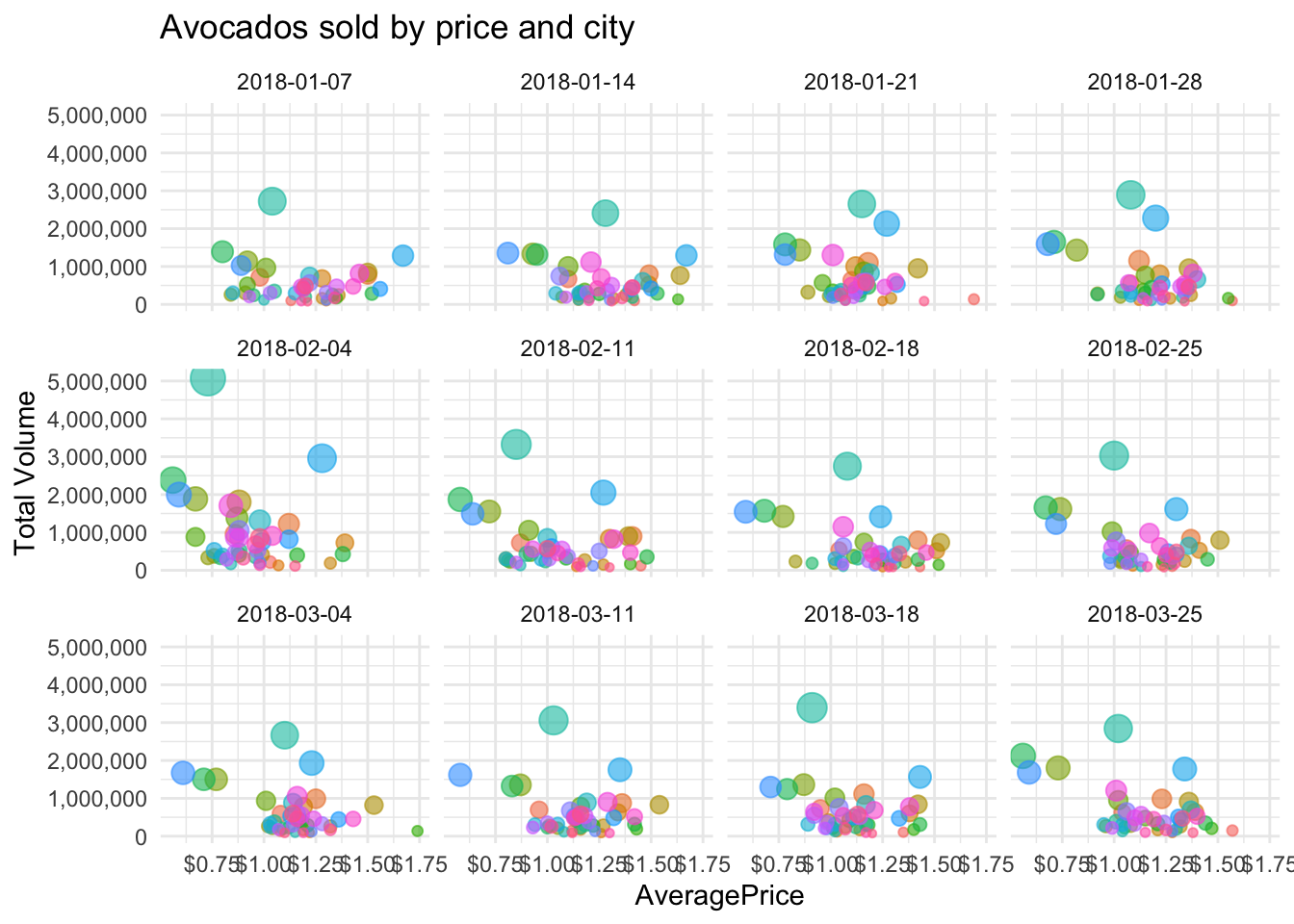Now we can move on to animating this. transition_time() will replace the previous dot, making it hard to see any trends. Let’s add `shadow_wake` so we can see the direction between points.

One really cool trick I like to employ is writing in the subtitle what point in time we’re currently animating. Before it didn’t really matter because the date was on the x axis, but not its hidden. For that, we need to add some `{}` in the subtitle argument of labs.

``````ggplot(data = avocado_cities_filtered,
mapping = aes(x = AveragePrice, y = `Total Volume`, color = region)) +
scale_y_continuous(labels = scales::comma_format()) +
scale_x_continuous(labels = scales::dollar_format()) +
geom_point(aes(size = `Total Volume`), alpha = .6) +
theme_minimal() +
theme(legend.position = "none") +
labs(title = "Avocados sold by price and city",
subtitle = "Date: {frame_time}") +
transition_time(Date) +

Let’s also save this, since each time we run the code it takes some time.

``anim_save(filename = "type_time.gif")``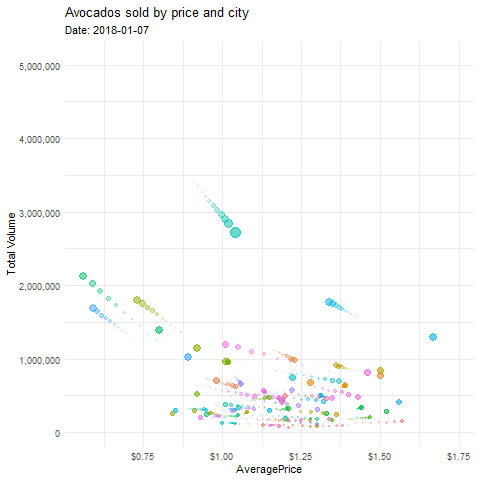### Challenge

Can you use transition_time to show how the price of organic avocados change over time for California?

## transition_state()

Transition_state() creates a new animation layer across a categorical variable instead of over time.

``````avocado_region_long <- avocado_region %>%
pivot_longer(cols = c(`4046`,`4225`,`4770`),
names_to = "size",
values_to = "volume")
mapping = aes(x = size, y = volume, color = size)) +
geom_boxplot() ``````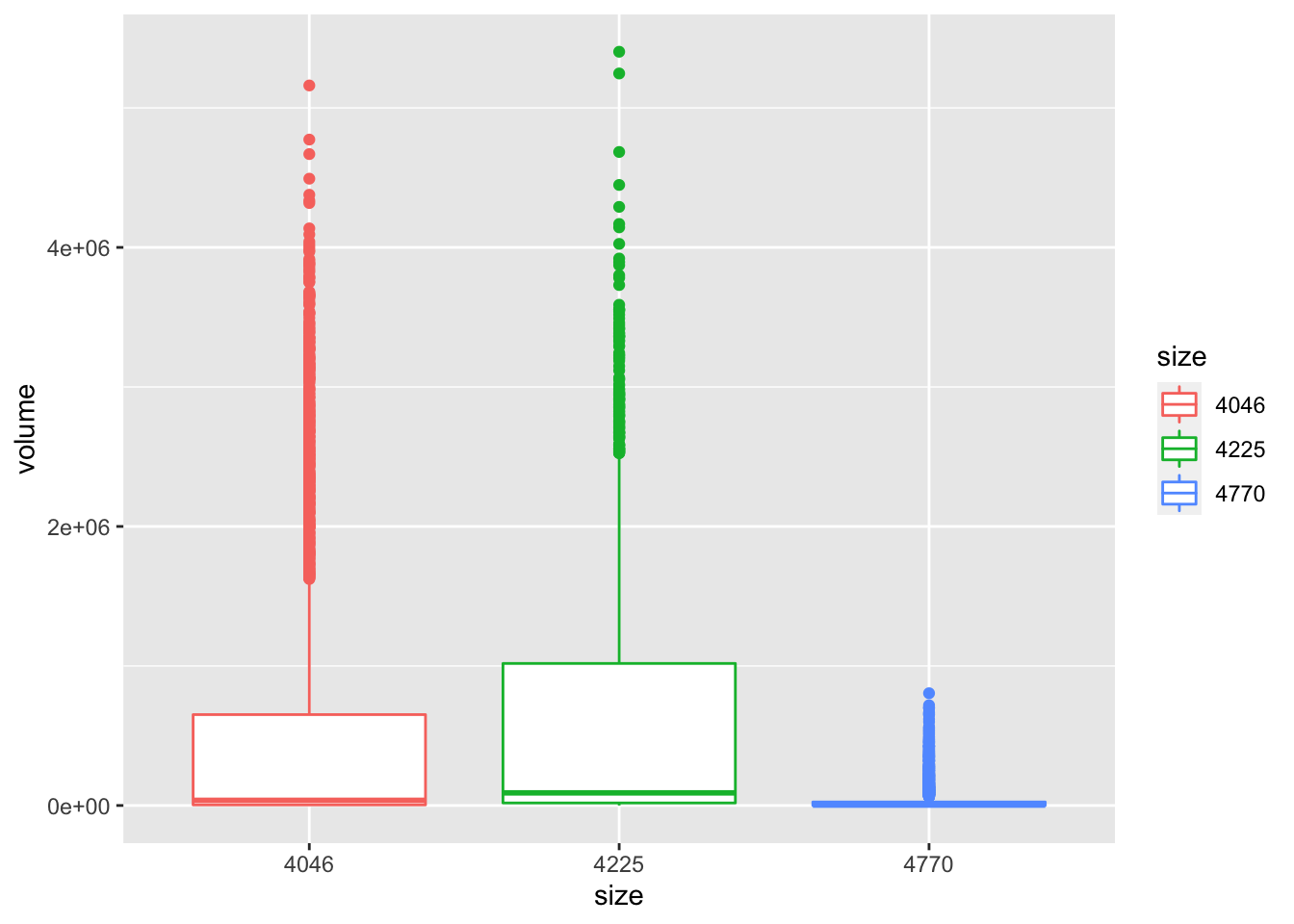Let’s customize this a little to make it look nicer.

``````ggplot(data = avocado_region_long,
mapping = aes(x = size, y = volume, color = size)) +
geom_boxplot() +
theme_classic() +
scale_y_continuous(labels = scales::comma_format()) +
labs(title = "Boxplot of volume sold by Avocado Size")``````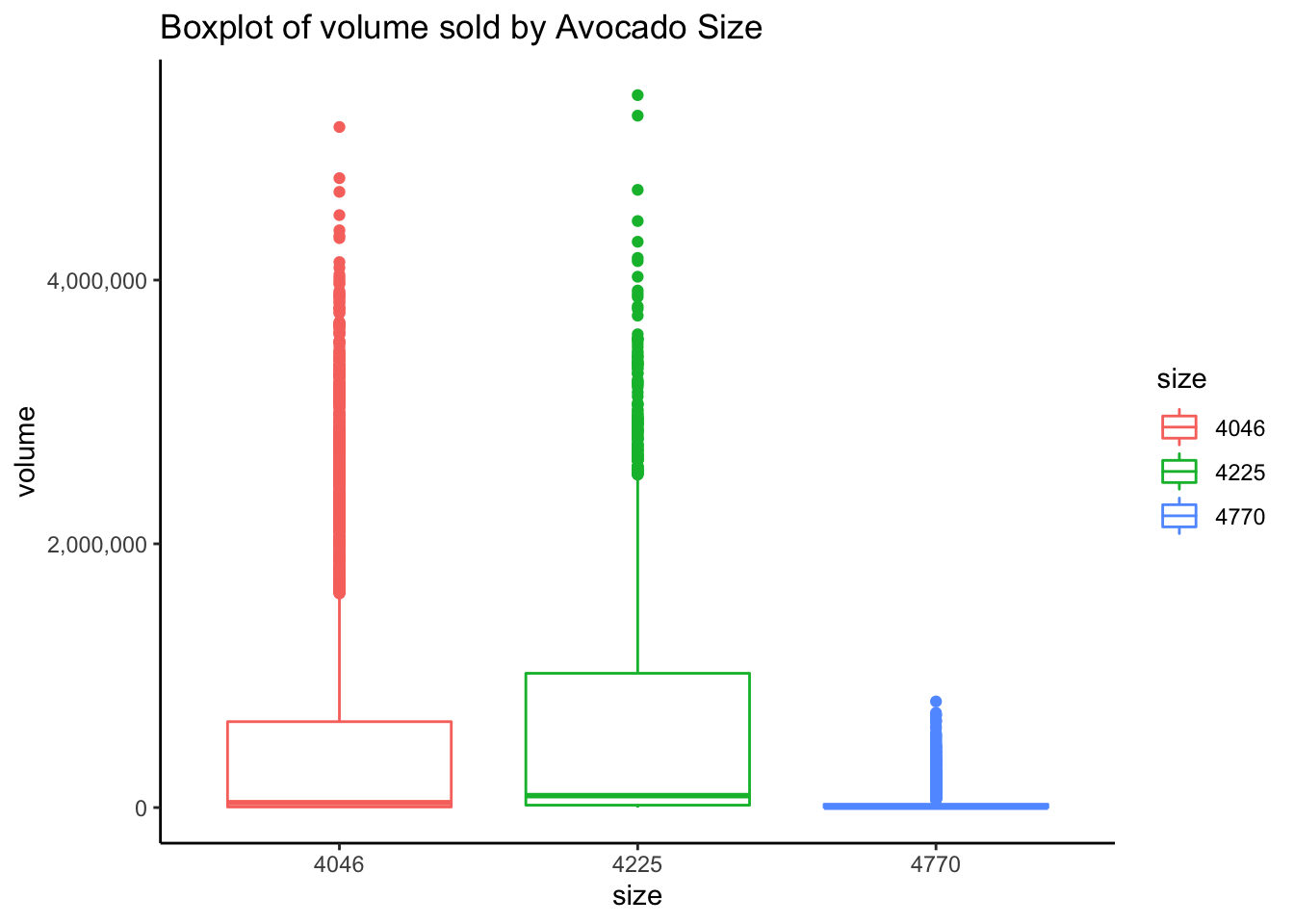It isn’t particularly helpful that the previous view completely dissappears as in transition_time. Instead of using `shadow_wake()`, let’s use `shadow_mark()` to the animated plot to keep the past views visible.

``````ggplot(data = avocado_region_long,
mapping = aes(x = size, y = volume, color = size)) +
geom_boxplot() +
theme_classic() +
scale_y_continuous(labels = scales::comma_format()) +
labs(title = "Boxplot of volume sold by Avocado Size") +
transition_states(size, state_length = 1, transition_length = 1) +
shadow_mark(alpha = 0.3, size = 0.5)    ``````

Let’s also save this, since each time we run the code it takes some time.

``anim_save(filename = "type_state.gif")``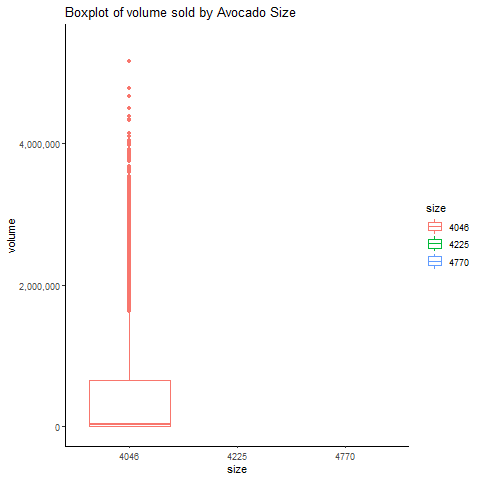Challenge 1: Take a few minutes to try and plot the changes in total volume across time for the different regions of the USA.

``````ggplot(data = filter(avocado_region, type == "organic"),
aes(x = Date, y = `Total Volume`, color = region)) +
geom_line() +
theme_minimal() +
labs(title = "Average Price of US Avocados",
caption = "Source: Kaggle",
subtitle = "Date: {frame_along}") +
transition_reveal(Date)
anim_save("challenge_1.gif")``````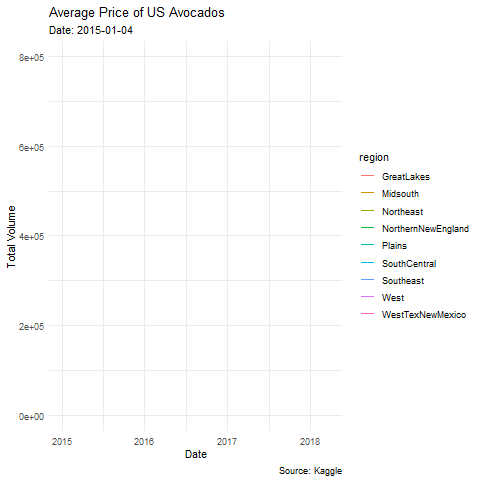Challenge 2: Can you use transition_time to show how the price of organic avocados change over time for California?

``````ggplot(data = filter(avocado_CA, type == "organic"),
mapping = aes(x = Date, y = AveragePrice)) +
scale_y_continuous(labels = scales::dollar_format()) +
geom_point(alpha = .6) +
theme_minimal() +
theme(legend.position = "none") +
labs(title = "The fluctuating price of organic avocados in California",
subtitle = "Date: {frame_time}") +
transition_time(Date) +